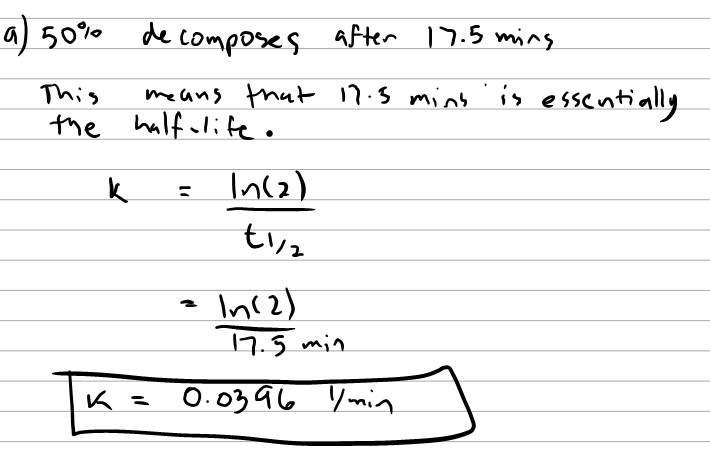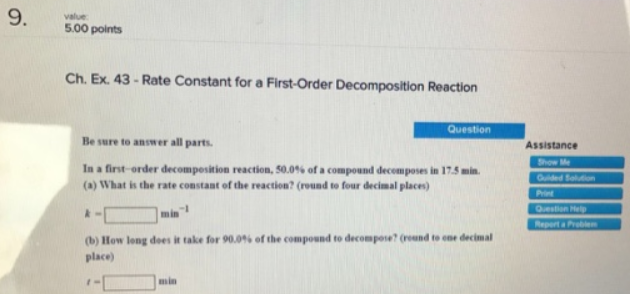# Problem: Rate Constant for a First-Order Decomposition Reaction Be sure to answer all parts.In a first-order decomposition reaction, 50.0% of a compound decomposes in 17.5 min. (a) What is the rate constant of the reaction? (round to four decimal places) k = _________ min-1 (b) How long does it takes for 90.0% of the compound to decompose? (round to one decimal place)

###### FREE Expert Solution84% (412 ratings)###### Problem Details

Rate Constant for a First-Order Decomposition Reaction

Be sure to answer all parts.

In a first-order decomposition reaction, 50.0% of a compound decomposes in 17.5 min.

(a) What is the rate constant of the reaction? (round to four decimal places)

k = _________ min-1

(b) How long does it takes for 90.0% of the compound to decompose? (round to one decimal place)